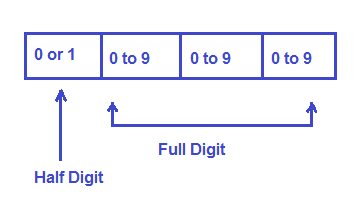# What is 3.5 or 4.5 Digit Meter Display?

If you go through the catalog of any instrument having digital display, you may notice that its display is given like three and half (3½) or four and half (4½) LCD display. So what does this mean or what information does this provide?

Basically, there are two types of digits in digital display: Full digit and Half Digit. A full digit is something which can take any value from 0 to 9. Thus it can have a total of 10 different states. This mean a full digit may have any value 0, 1, 2,….., or 9. A half digit is something which can either have a value of 0 or 1. This digit is basically the most significant digit and hence has limited use for displaying any number or reading.

Let us now understand the meaning of 3.5 and 4.5 digit digital readout.

### Meaning of 3½Digit Display

3.5 digit displays have four digits: one half digit and three full digits. As half digit is the most significant digit and can either have vale 0 or 1 and full digit can take any value (0 to 9), therefore the range of digital display will be 0 to 1999.Since negative sign can be displayed by the readout, the range will be -1999 to 1999. If we want to display 30 on 3.5 digit display, we need to throw out the half digit. The three full digits will be used. Thus the value displayed will be 300. Now we need to place a decimal. Decimal point in digital display is just a dot symbol which is manually displayed after appropriate number of segment to show the complete desired number. This means that the displayed value will be 30.0

### Meaning of 4½ Digit Display

4.5 digit display have five digits: 1 half digit and 4 full digit. Thus as discussed in 3.5 digit display, the range of the display will be 0 to 19999. As negative sign can also be displayed by the instrument, the range will be -19999 to 19999.

### Meaning of 3¾ Digit Display

In 3¾ digit display, the most significant digit may either be 0,1,2 or 3. While the three full digits may have any value from 0 to 9. Thus the maximum value displayed by such digital readout is 3999. The range of displayed value will be -3999 to 3999.

This site uses Akismet to reduce spam. Learn how your comment data is processed.